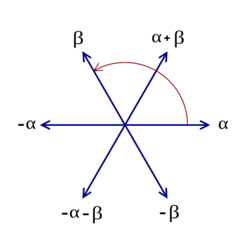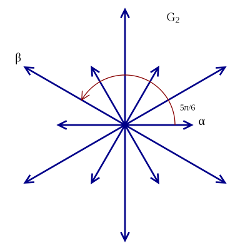I’ve been re-reading parts of Carter’s “Finite groups of Lie type” and Malle-Testerman’s “Linear algebraic groups and finite groups of Lie type” with a view to understanding the theory of maximal tori in finite groups of Lie type.

In this post I want to use the theory in those books to write down the orders of the maximal tori of $A_2(q)$, ${^2A_2(q)}$ and $G_2(q)$. I wanted to also do ${^2G_2(q)}$ but, so far, I haven’t managed to write things down properly for the Ree and Suzuki groups, so I’ll exclude these from what follows.

The general set-up is as follows: $G$ is a simple linear algebraic groups, and $F:G\to G$ is a Steinberg endomorphism, i.e. some power $F^m:G\to G$ is a Frobenius endomorphism of $G$. (Some of this theory works more generally – for $G$ connected reductive – and, in particular, this can be important when one studies centralizers inside a simple LAG’s…. But I’m not going there just now.) Now a theorem of Steinberg asserts that $G^F$, the set of fixed-points of $F$, is a finite set [MT, Theorem 21.5] – such a group is an example of a finite group of Lie type.

Now the Lang-Steinberg theorem asserts that the map $L:G\to G, \, g\mapsto F(g) g^{-1}$ is surjective [MT, Theorem 21.7]. This theorem then implies that $G$ contains an $F$-stable maximal torus $T$ inside an $F$-stable Borel subgroup $B$. Since $T$ is $F$-stable, $N_G(T)$ is also $F$-stable, and so $F$ naturally acts on the Weyl group $W=N_G(T)/T$ of $G$. Similarly, $F$ acts on the character group $X:=X(T)$ via

$F(\chi(t)):= \chi(F(t)) \textrm{ for } \chi \in X, t\in T.$

We will need the notion of $F$-conjugacy in $W$: if $w_1, w_2\in W$, then $w_1$ is $F$-conjugate with $w_2$ if there exists $g\in W$ such that $w_1=F(g)w_2g^{-1}$.

Let $\Phi\subset X$ be the root system of $G$ with positive system $\Phi^+$ with respect to $T$ and $B$. In what follows we write $X_\mathbb{R}:=X\otimes_{\mathbb{Z}}\mathbb{R}$.

Now the first three results of [MT, Section 22.1] imply that

• there exists a natural number $\delta$ such that $F^\delta=r.1$ in its action on $X$, where $r$ is some power of $p$;
• there exists a permutation $\rho$ of $\Phi^+$ such that, for each $\alpha\in\Phi^+$, $F(\rho(\alpha)) = q_\alpha \alpha$ where $q_\alpha>1$ is a power of $p$;
• the parameter $q_\alpha$ is constant on root lengths; moreover, either $q_\alpha=q$ or else $(G,p)\in{(B_2,2), (G_2,3), (F_4,2)}$, $\rho$ interchanges long and short roots, and $q_{long}.q_{short}=q^2$ with $q_{short}/q_{long}=p$;
• setting $q=r^{1/\delta}$, we have that $F=q\phi$, where $q$ is the Frobenius endomorphism, and $\phi\in {\rm Aut}(X_\mathbb{R})$ is of order $\delta$ inducing $\rho^{-1}$ on $\Phi^+$;
• $T^F=X/(F-1)/X$.

It is important to note that, in principle, $q$ is a fractional power of $p$ (although, in fact, it will be integral except when $G^F$ is Ree or Suzuki). Note, too, that this set-up clearly defines the real number $q$ to be associated to our finite group of Lie type – for certain families (e.g. the unitaries), the value of $q$ follows varying conventions whereas here it is clear cut.

We have set-up all the necessary parameters associated with our group of Lie type. Now let’s study the maximal tori: we follow [MT, Chapter 25]. First off, we note that, since Frobenius endomorphism commute with elements of $W$ in their action on $T$, the notion of $F$-conjugacy is the same as $\phi$-conjugacy (where $F=q\phi$).

The following principles are important:

• [MT, Prop. 25.1] The $G^F$-classes of $F$-stable maximal tori of $G$ are in 1-1 correspondence with the $\phi$-conjugacy classes in $W$.
• [MT, Exercise 30.5] The $G^F$-classes of subgroups of the form $T^F$ ($T$ an $F$-stable maximal torus of $G$) are in 1-1 correspondence with the two previous sets. (I’m slightly unsure of this… But it seems correct.)

These correspondences follow from the Lang-Steinberg theorem. More precisely the first correspondence is as follows: if $gTg^{-1}$ is $F$-stable, then it corresponds to the element $w:=g^{-1}F(g)T\in N_g(T)/T=W$. We are then able to write $T_w$ for the conjugate $gTg^{-1}$. Note that $T_1$ corresponds to an $F$-stable maximal torus in an $F$-stable Borel subgroup. Now [MT, Prop. 25.3] asserts:

• $T_w^F\cong X/(wF-1)X$;
• $|T_w^F|=|\det_{X\otimes \mathbb{R}}(wF-1)|=\det_{X\otimes \mathbb{R}}(q-(w\phi)^{-1})$.

Specific calculations now follow. These can be confirmed using Kantor-Seress “Prime power graphs for groups of Lie type”.

## Calculations for $A_2(q)$

We record the size of the maximal tori for $A_2(q)$. Note that, here and below, the isogeny class does not matter – so, in this case, these calculations are valid for ${\rm PGL}_3(q)$ and ${\rm SL}_3(q)$.We use the fact that the fundamental roots of $A_2$ – labelled $\alpha$ and $\beta$ in the diagram – form a basis for $X\otimes\mathbb{R}$. With respect to this basis we have $$q=\left(\begin{matrix} q & 0 \\ 0 & q \end{matrix}\right).$$ In this case $\phi$ is trivial, so we just need to write down $q-w^{-1}$. The possibilities are as follows:

• $w=1$. Then $$w=\left(\begin{matrix} 1 & 0 \\ 0 & 1\end{matrix}\right)$$ and
$\det(q-w^{-1})=\det\left(\begin{matrix} q-1 & 0 \\ 0 & q-1\end{matrix}\right) =(q-1)^2.$
• $w=Ref_\alpha$. Then $$w=\left(\begin{matrix} -1 & 1\\ 0 & 1\end{matrix}\right)$$ and
$\det(q-w^{-1})=\det\left(\begin{matrix} q+1 & -1 \\ 0 & q-1\end{matrix}\right) = q^2-1.$
• $w=Rot_{\pi/3}$. Then $$w=\left(\begin{matrix} 0 & -1\\ 1 & -1\end{matrix}\right)$$ and
$\det(q-w^{-1}=\det\left(\begin{matrix} q & 1 \\ -1 & q+1\end{matrix}\right) = q^2+q+1.$

## Calculations for ${^2A_2}(q)$

We record the size of the maximal tori for ${^2A_2}(q)$. The root system is as before, and we have the same value for $q$, but this time time $\phi$ is non-trivial.

Using the same basis as before – ${\alpha, \beta}$, we can write $\phi$ as $$\left(\begin{matrix} 0 & 1 \\ 1 & 0 \end{matrix}\right)$$. This is just taking $\phi$ acting on the Dynkin diagram. (Stupid comment: I’ve never cottoned on to the fact, hitherto, that $\phi$ is also an automorphism of the root system. In particular it normalizes the Weyl group which here is $W\cong D_6$. So we get $\langle W, \phi\rangle \cong D_{12}$. This is clearly true in general.)

So now we need to write down $q-(\phi w)^{-1}$. The possibilities for $w$ are as before:

• $w=1$. Then $$w=\left(\begin{matrix} 1 & 0 \\ 0 & 1\end{matrix}\right)$$ and
$\det(q-(\phi w)^{-1})=\det\left(\begin{matrix} q & 1 \\ 1 & q\end{matrix}\right) = q^2-1.$
• $w=Ref_\alpha$. Then $$w=\left(\begin{matrix} -1 & 1\\ 0 & 1\end{matrix}\right)$$ and
$\det(q-(\phi w)^{-1})=\det\left(\begin{matrix} q-1 & 1 \\ -1 & q\end{matrix}\right) = q^2-q+1.$
• $w=Ref_{\alpha+\beta}$. Then $$w=\left(\begin{matrix} 0 & -1\\ -1 & 0\end{matrix}\right)$$ and
$\det(q-(\phi w)^{-1})=\det\left(\begin{matrix} q+1 & 0 \\ 0 & q+1\end{matrix}\right) = (q+1)^2.$

Note that we need to choose different elements $w$ because the $\phi$-conjugacy classes in $W$ are different to the usual conjugacy classes.

## Calculations for $G_2(q)$

Recall that $G_2(q)$ has order $q^6(q^2-1)(q^2-1)$.As before, we take ${\alpha, \beta}$ as a basis for $X\otimes\mathbb{R}$, and we note that $\phi$ is trivial, and $q$ is as before. We must go through representatives for each of the conjugacy classes of $W=D_{12}$:

• $w=1$. Then $$w=\left(\begin{matrix} 1 & 0 \\ 0 & 1\end{matrix}\right)$$ and
$\det(q-w^{-1})=\det\left(\begin{matrix} q-1 & 0 \\ 0 & q-1\end{matrix}\right) =(q-1)^2.$
• $w=-1=Rot_{\pi/2}$. Then $$w=\left(\begin{matrix} -1 & 0\\ 0 & -1\end{matrix}\right)$$ and
$\det(q-w^{-1})=\det\left(\begin{matrix} q+1 & 0 \\ 0 & q+1\end{matrix}\right) = (q+1)^2.$
• $w=Rot_{\pi/3}$. Then $$w=\left(\begin{matrix} 1 & -3\\ 1 & -2\end{matrix}\right)$$ and
$\det(q-w^{-1})=\det\left(\begin{matrix} q-1 & -1 \\ 3 & q+2\end{matrix}\right) = q^2+q+1.$
• $w=Rot_{\pi/6}$. Then $$w=\left(\begin{matrix} 2 & -3\\ 1 & -1\end{matrix}\right)$$ and
$\det(q-w^{-1})=\det\left(\begin{matrix} q-2 & 3 \\ -1 & q+1\end{matrix}\right) = q^2-q+1.$
• $w=Ref_{3\alpha+2\beta}$. Then $$w=\left(\begin{matrix} 1 & -3\\ 0 & -1\end{matrix}\right)$$ and
$\det(q-w^{-1})=\det\left(\begin{matrix} q-1 & 3 \\ 0 & q+1\end{matrix}\right) = q^2-1.$

This yields all of the maximal tori that are listed in Kantor-Seress. However note that there are two conjugacy classes of reflections in $D_{12}$ – here they correspond to reflections in long and short roots – and so we obtain another example:

• $w=Ref_{\alpha+\beta}$. Then $$w=\left(\begin{matrix} 2 & -3\\ 1 & -2\end{matrix}\right)$$ and
$\det(q-w^{-1})=\det\left(\begin{matrix} q-2 & 3 \\ -1 & q+2\end{matrix}\right) = q^2-1.$

Thus it appears that there are two conjugacy classes of maximal torus of order $q^2-1$ – I guess one occurs as a split torus in a Levi factor ${\rm GL}_2(q)=\langle U_\alpha, U_{-\alpha}\rangle$, while the other occurs in ${\rm GL}_2(q)=\langle U_\beta, U_{-\beta}\rangle$. I reconcile this to Kantor-Seress by noting that they do not necessarily claim to list all conjugacy classes of tori, although in some places they do note that there is more than one conjugacy class of a certain order.